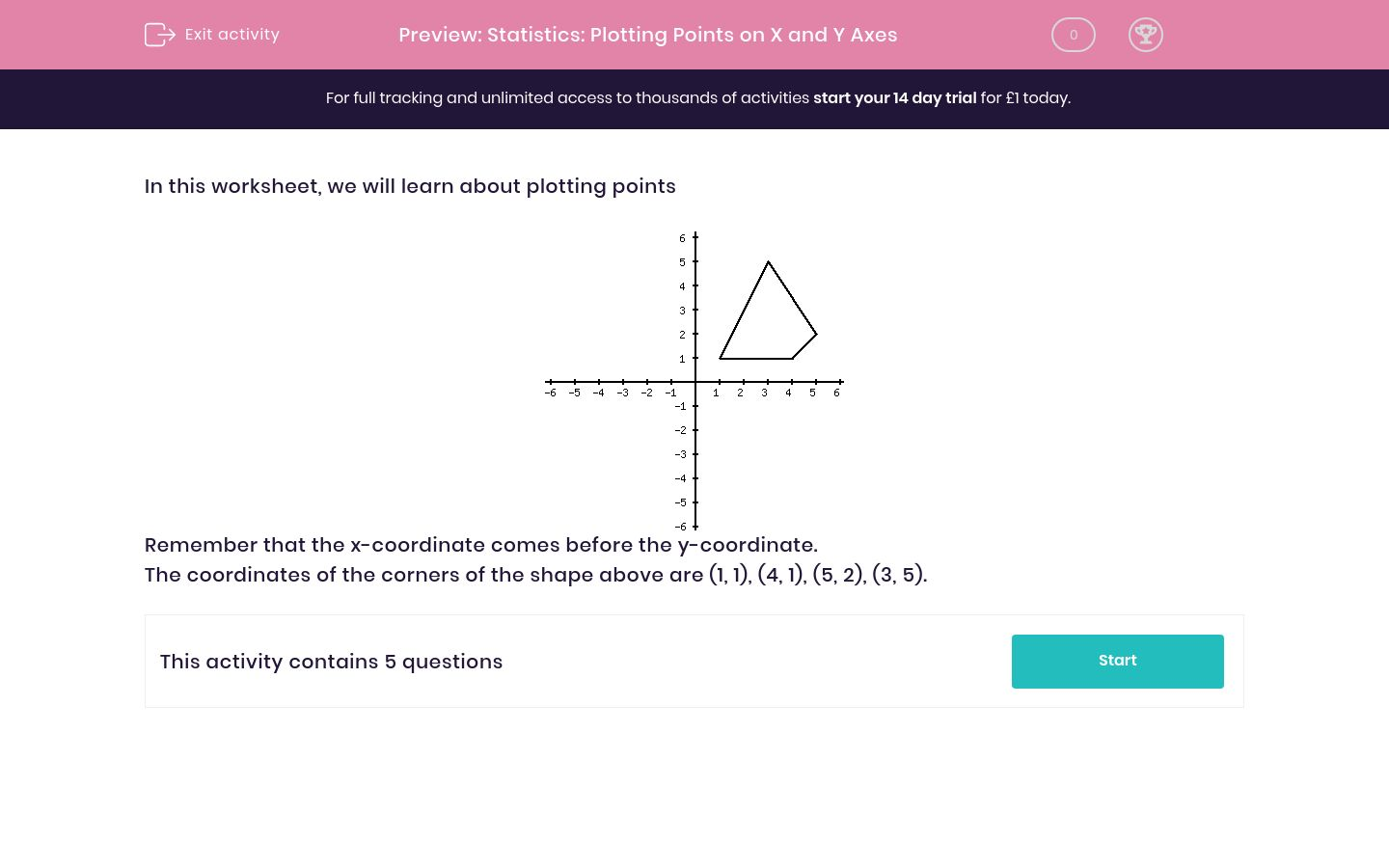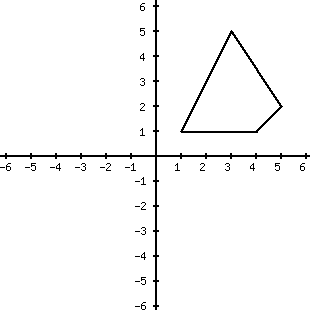# Statistics: Plotting Points on X and Y Axes

In this worksheet, students plot and read points and understand the simple reflection of points in the x- and y-axes.Key stage:  KS 2

Curriculum topic:   Maths and Numerical Reasoning

Curriculum subtopic:   Interpreting Data

Difficulty level:### QUESTION 1 of 10

In this worksheet, we will learn about plotting pointsRemember that the x-coordinate comes before the y-coordinate.

The coordinates of the corners of the shape above are (1, 1), (4, 1), (5, 2), (3, 5).

Plot these points and join them as you go. (1, 1)(4, 1)(5, 2)(3, 5).
Which shape will you obtain?  A, B, C, or DA

B

C

D

Plot these points and join them as you go. (1, 1)(4, 1)(5, 2)(3, 5).
Which shape you will obtain on reflecting your shape in the y-axis (A, B, C, D)?A

B

C

D

Plot these points and join them as you go. (1, 1)(4, 1)(5, 2)(3, 5).
Which shape you will obtain on reflecting your shape in the x-axis (A, B, C, D)?A

B

C

D

Plot these points and join them as you go. (1, 1)(4, 1)(5, 2)(3, 5).
What co-ordinates will you obtain on reflecting your shape in the y-axis?(-1, 1), (-4, 1), (-5, 2), (-3, 5)

(1, -1), (4, -1), (5, -2), (3, -5)

Plot these points and join them as you go. (1, 1)(4, 1)(5, 2)(3, 5).
What co-ordinates will you obtain on reflecting your shape in the x-axis?(-1, 1), (-4, 1), (-5, 2), (-3, 5)

(1, -1), (4, -1), (5, -2), (3, -5)

• Question 1

Plot these points and join them as you go. (1, 1)(4, 1)(5, 2)(3, 5).
Which shape will you obtain?  A, B, C, or DB
• Question 2

Plot these points and join them as you go. (1, 1)(4, 1)(5, 2)(3, 5).
Which shape you will obtain on reflecting your shape in the y-axis (A, B, C, D)?A
• Question 3

Plot these points and join them as you go. (1, 1)(4, 1)(5, 2)(3, 5).
Which shape you will obtain on reflecting your shape in the x-axis (A, B, C, D)?C
• Question 4

Plot these points and join them as you go. (1, 1)(4, 1)(5, 2)(3, 5).
What co-ordinates will you obtain on reflecting your shape in the y-axis?(-1, 1), (-4, 1), (-5, 2), (-3, 5)
EDDIE SAYS
Remember x comes before y in coordinates
• Question 5

Plot these points and join them as you go. (1, 1)(4, 1)(5, 2)(3, 5).
What co-ordinates will you obtain on reflecting your shape in the x-axis?(1, -1), (4, -1), (5, -2), (3, -5)
---- OR ----

Sign up for a £1 trial so you can track and measure your child's progress on this activity.

### What is EdPlace?

We're your National Curriculum aligned online education content provider helping each child succeed in English, maths and science from year 1 to GCSE. With an EdPlace account you’ll be able to track and measure progress, helping each child achieve their best. We build confidence and attainment by personalising each child’s learning at a level that suits them.

Get started